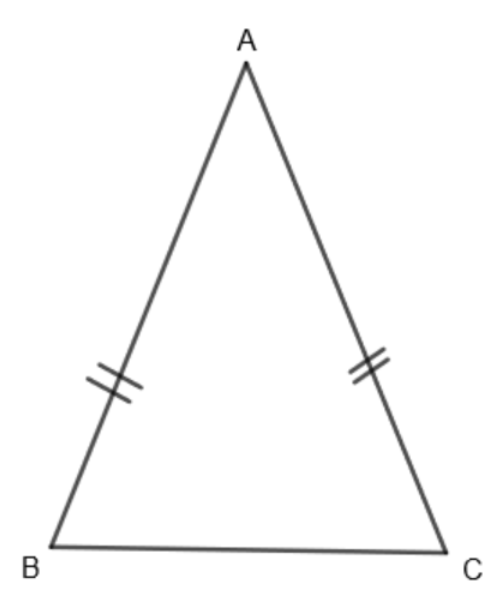Courses
Courses for Kids
Free study material
Offline Centres
MoreLast updated date: 05th Dec 2023
Total views: 381.9k
Views today: 9.81k

# The base of an isosceles triangle is $\dfrac{4}{3}\text{cm}$. The perimeter of the triangle is $\dfrac{62}{15}\text{cm}$. What is the length of either of the remaining equal sides?Verified
381.9k+ views

Hint: In this question, the perimeter and length of the base are given. Take the sum of the sides of the isosceles triangle and equate it to the perimeter. Then substitute the given values to find the length of the two remaining sides.

Here, we are given that the base of the isosceles triangle is $\dfrac{4}{3}\text{cm}$. The perimeter of the triangle is $\dfrac{62}{15}\text{cm}$. We need to find the length of either of the remaining sides.

Before proceeding with this question, we must know what an isosceles triangle is. An isosceles triangle is a triangle that has two sides of equal length. The two sides of triangles are called legs while the third side is called the base of the triangle.

Let us consider an isosceles triangle ABC.In the above isosceles triangle, sides AB = AC and side BC is the third side that is the base of the given triangle.

Now, we know that the perimeter of any polygon is given by the sum of its sides. So, we get,

Perimeter of the triangle = Sum of its 3 sides

Therefore, for the given triangle, we get,

Perimeter of $\Delta \text{ABC = AB + AC + BC}$

We know that AB = AC.

So, let us substitute AB = AC = x. So, we get,

Perimeter of $\Delta \text{ABC = 2x + BC}$

Also, we know that BC is the base of the triangle. So, we get,

Perimeter of isosceles $\Delta \text{ABC = 2x + Base of }\Delta \text{ABC}$

So, for any general isosceles triangle, we get,

Perimeter of isosceles triangle = 2 (Length of either of the equal sides) + (length of the base)

We are given that the base of the isosceles triangle $=\dfrac{4}{3}\text{cm}$ and its perimeter $=\dfrac{62}{15}\text{cm}$. By substituting these values in the above equation, we get,

$\dfrac{62}{15}\text{cm = 2 }\left( \text{length of either of equal sides} \right)+\text{ }\dfrac{4}{3}\text{cm}$

By subtracting $\dfrac{4}{3}$ from both the sides of the above equation, we get,

$\dfrac{62}{15}-\dfrac{4}{3}=2\left( \text{length of either of equal sides} \right)$

$\Rightarrow \dfrac{62-20}{15}=2\left( \text{length of either of equal sides} \right)$

Or, $2\left( \text{length of either of equal sides} \right)=\dfrac{42}{15}$

Now by dividing 2 on both the sides, we get,

Length of either of equal side $=\dfrac{7}{5}\text{cm}\approx \text{1}\text{.4cm}$

Hence, we get the length of each equal side of the isosceles triangle as $\dfrac{7}{5}$ or 1.4 cm.

Note:

Students must note the triangles are divided into 3 categories based on length of sides that are scalene triangles, isosceles triangles and equilateral triangles. In scalene triangles, all sides are unequal, in isosceles triangles, at least 2 out of 3 sides are equal while in the equilateral triangle, all sides are equal. Students can also cross-check their answer by adding the length of all the sides and equating it to the given perimeter and checking if LHS = RHS or not.